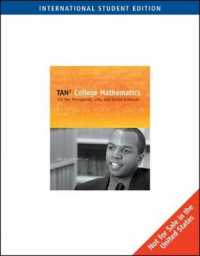### College Mathematics for the Managerial, Life, and Social Sciences, International Edition -- Paperback / softback （Internatio）

• ただいまウェブストアではご注文を受け付けておりません。 ⇒古書を探す
• 製本 Paperback:紙装版/ペーパーバック版
• 商品コード 9780495119722

### Full Description

This text helps you succeed in finite mathematics and applied calculus by using clear explanations, real-life examples, and up-to-date technology. Real-life applications-such as satellite radio subscriptions, Google's revenue, job outsourcing, and the effects of smoking bans-are drawn from the areas of business and the behavioral, life, and social sciences. Portfolio profiles give you a firsthand look at how real-world professionals use finite mathematics and applied calculus in their work. You can also take advantage of extensive online support to enhance your learning, including video instruction and interactive tutorials that walk you step by step through examples and problems in the text.

### Contents

1. STRAIGHT LINES AND LINEAR FUNCTIONS.The Cartesian Coordinate System. Straight Lines. Linear Functions and Mathematical Models. Intersection of Straight Lines. The Method of Least Squares.Systems of Linear Equations: An Introduction. Systems of Linear Equations: Unique Solutions. Systems of Linear Equations: Underdetermined and Overdetermined Systems. Matrices. Multiplication of Matrices. The Inverse of a Square Matrix. Leontief Input-Output Model.3. LINEAR PROGRAMMING: A GEOMETRIC APPROACH.Graphing Systems of Linear Inequalities in Two Variables. Linear Programming Problems. Graphical Solution of Linear Programming Problems. Sensitivity Analysis.4. LINEAR PROGRAMMING: AN ALGEBRAIC APPROACH.The Simplex Method: Standard Maximization Problems. The Simplex Method: Standard Minimization Problems. The Simplex Method: Nonstandard Problems.Compound Interest. Annuities. Amortization and Sinking Funds. Arithmetic and Geometric Progressions.6. SETS AND COUNTING.Sets and Set Operations. The Number of Elements in a Finite Set. The Multiplication Principle. Permutations and Combinations.7. PROBABILITY.Experiments, Sample Spaces, and Events. Definition of Probability. Rules of Probability. Use of Counting Techniques in Probability. Conditional Probability and Independent Events. Bayes'' Theorem.8. PROBABILITY DISTRIBUTIONS AND STATISTICS.Distributions of Random Variables. Expected Value. Variance and Standard Deviation. The Binomial Distribution. The Normal Distribution. Applications of the Normal Distribution.Markov Chains. Regular Markov Chains. Absorbing Markov Chains.10. PRECALCULUS REVIEW.Exponents and Radicals. Algebraic Expressions. Algebraic Fractions. Inequalities and Absolute Values.11. FUNCTIONS, LIMITS, AND THE DERIVATIVE.Functions and Their Graphs. The Algebra of Functions. Functions and Mathematical Models. Limits. One-Sided Limits and Continuity. Derivative.12. DIFFERENTIATION.Basic Rules of Differentiation. The Product and Quotient Rules. The Chain Rule. Marginal Functions in Economics. Higher-Order Derivatives. Implicit Differentiation and Related Rates. Differentials.Applications of the First Derivative. Applications of the Second Derivative. Curve Sketching. Optimization I. Optimization II.14. EXPONENTIAL AND LOGARITHMIC FUNCTIONS.Exponential Functions. Logarithmic Functions. Differentiation of Exponential Functions. Differentiation of Logarithmic Functions. Exponential Functions as Mathematical Models.15. INTEGRATION.Antiderivatives and the Rules of Integration. Integration by Substitution. Area and the Definite Integral. The Fundamental Theorem of Calculus. Evaluating Definite Integrals. Area Between Two Curves. Applications of the Definite Integral to Business and Economics.Integration by Parts. Integration Using Tables of Integrals. Numerical Integration. Improper Integrals. Applications of Calculus to Probability.Functions of Several Variables. Partial Derivatives. Maxima and Minima of Functions of Several Variables. The Method of Least Squares. Constrained Maxima and Minima and the Method of Lagrange Multipliers. Double Integrals.Appendix A: The System of Real Numbers.Appendix B: Tables.Answers to Odd-Numbered Exercises.Index.